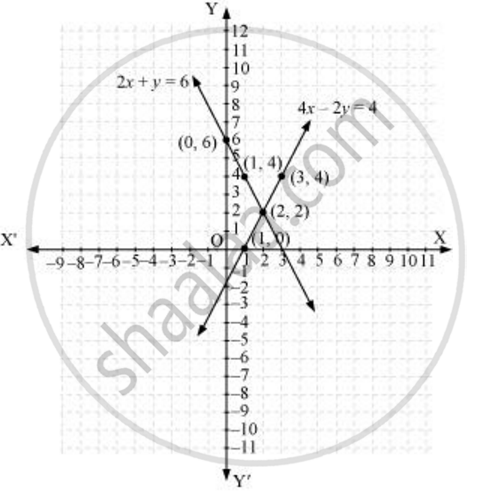# Which of the following pairs of linear equations are consistent/ inconsistent? If consistent, obtain the solution graphically: 2x + y – 6 = 0, 4x – 2y – 4 = 0 - Mathematics

Which of the following pairs of linear equations are consistent/ inconsistent? If consistent, obtain the solution graphically: 2x + y – 6 = 0, 4x – 2y – 4 = 0

#### Solution

2x + y - 6 = 0

4x - 2y - 4 = 0

a_1/a_2 = 2/4 = 1/2, b_1/b_2 = (-1)/2 and c_1/c_2 = (-6)/-4 = 3/2

Since a_1/a_2 != b_1/b_2

Therefore, these linear equations are intersecting each other at one point and thus have only one possible solution. Hence, the pair of linear equations is consistent.

2x + y − 6 = 0

y = 6 − 2x

 x 0 1 2 y 6 4 2

And 4− 2y − 4 = 0

y = (4x - 4)/2

 x 1 2 3 y 0 2 4

Hence, the graphic representation is as follows.From the figure, it can be observed that these lines are intersecting each other at the only point i.e., (2, 2) and it is the solution for the given pair of equations.

Concept: Graphical Method of Solution of a Pair of Linear Equations
Is there an error in this question or solution?
Chapter 3: Pair of Linear Equations in Two Variables - Exercise 3.2 [Page 50]

#### APPEARS IN

NCERT Class 10 Maths
Chapter 3 Pair of Linear Equations in Two Variables
Exercise 3.2 | Q 4.3 | Page 50
Share